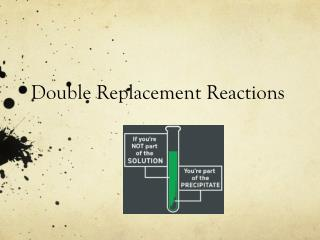DownloadDownload PresentationDouble Replacement Reactions

# Double Replacement Reactions

Télécharger la présentation## Double Replacement Reactions

- - - - - - - - - - - - - - - - - - - - - - - - - - - E N D - - - - - - - - - - - - - - - - - - - - - - - - - - -
##### Presentation Transcript

1. Double Replacement Reactions

2. Double Replacement Reactions • Double Replacement Reactions occur when a metallic ion replaces another metallic ion in a compound and a nonmetal replaces a nonmetal in a compound, or when an acid reacts with a base. • Compound + compound  new compound+ new compound • AX + BY  AY + BX • A precipitate (solid), a gas or water forms.

3. Review: Phases of matter • (s) solid, like copper metal • (aq) aqueous = dissolved in water, like salt water • (l) liquid, like water, gasoline • (g) gas, like carbon dioxide, oxygen

4. Precipitate • A solid that forms when two aqueous ionic compounds react. • Label the precipitates with (s)

5. Double Replacement Reactions • Think about it like “foil”ing in algebra, first and last ions go together + inside ions go together • Example: AgNO3(aq) + NaCl(aq)  AgCl(s) + NaNO3(aq) • Another example: K2SO4(aq) + Ba(NO3)2(aq)  KNO3(aq) + BaSO4(s) 2

6. Memorize These Acids • Sulfuric acid H2SO4 • Nitric Acid HNO3 • Phosphoric acid H3PO4 • Carbonic acid H2CO3 • Acetic acid HC2H3O2 (or: CH3COOH) • Hydrochloric acid HCl

7. Neutralization Reactions • An acid + Base  a salt and water • Example: Hydrochloric acid + sodium hydroxide  sodium chloride + water HCl(aq) + NaOH(aq)  NaCl(aq) + H2O(l)

8. Solubility Table

9. Practice • Predict the products. Balance the equation • HCl(aq) + AgNO3(aq)  • CaCl2(aq) + Na3PO4(aq)  • Pb(NO3)2(aq) + BaCl2(aq)  • FeCl3(aq) + NaOH(aq)  • H2SO4(aq) + NaOH(aq)  • KOH(aq) + CuSO4(aq) 

10. Answers: • HCl(aq) + AgNO3(aq)  AgCl(s) + HNO3(aq) • 3 CaCl2(aq) + 2Na3PO4(aq)  6 NaCl(aq) + Ca3(PO4)2(s) • Pb(NO3)2(aq) + BaCl2(aq)  PbCl2(s) + Ba(NO3)2(aq) • FeCl3(aq) + 3 NaOH(aq)  Fe(OH)3(s) + 3 NaCl(aq) • H2SO4(aq) + 2 NaOH(aq)  2 H2O(l) + Na2SO4(aq) • 2 KOH(aq) + CuSO4(aq)  K2SO4 (aq) + Cu(OH)2(s)

11. Total Ionic Equations • Once you write the molecular equation (synthesis, decomposition, etc.), you should check for reactants and products that are soluble or insoluble. • We usually assume the reaction is in water • We can use a solubility table to tell us what compounds dissolve in water. • If the compound is soluble (does dissolve in water), then splits the compound into its component ions • If the compound is insoluble (does NOT dissolve in water), then it remains as a compound

12. Total Ionic Equations Molecular Equation: K2CrO4 + Pb(NO3)2 PbCrO4 + 2 KNO3 Soluble Soluble Insoluble Soluble Total Ionic Equation: 2 K+ + CrO4-2 + Pb+2 + 2 NO3-  PbCrO4 (s) + 2 K+ + 2 NO3-

13. Spectator ions Spectator ions just “watch” the reaction occur. They remain the same before and after the reaction. In the previous reaction, potassium and nitrate ions are spectator ions. They do not change!

14. Net Ionic Equations • These are the same as total ionic equations, but you should cancel out ions that appear on BOTH sides of the equation Total Ionic Equation: 2 K+ + CrO4-2 + Pb+2 + 2 NO3-  PbCrO4 (s) + 2 K+ + 2 NO3- Net Ionic Equation: CrO4-2 + Pb+2  PbCrO4 (s)

15. Net Ionic Equations • Try this one! Write the molecular, total ionic, and net ionic equations for this reaction: Silver nitrate reacts with magnesium chloride. Equation: 2 AgNO3(aq) + MgCl2(aq)  Mg(NO3)2(aq) + 2 AgCl(s) Total Ionic: Net Ionic: